## Babyplots R Package

Babyplots is an easy to use library for creating interactive 3d graphs for exploring and presenting data.

### Usage

The babyplots R package can be installed from its bitbucket page using devtools:

devtools::install_bitbucket("derpylz/babyplots.git")

library(babyplots)

This will expose the six plotting functions:

pointCloud()
shapeCloud()
heatMap()
surface()
imgStack()
meshObject()

as well as the corresponding Shiny bindings

# pointCloud Shiny bindings:
pointCloudOutput()
renderPointCloud()
# shapeCloud Shiny bindings:
shapeCloudOutput()
renderShapeCloud()
# heatMap Shiny bindings:
heatMapOutput()
renderHeatmap()
# surface Shiny bindings:
surfaceOutput()
renderSurface()
# imgStack Shiny bindings:
imgStackOutput()
renderImgStack()
# meshObject Shiny bindings:
meshObjectOutput()
renderMeshObject()


You can remind yourself of the arguments to the plotting functions at any time using the R manual:

?pointCloud
?shapeCloud
?heatMap
?surface
?imgStack
?meshObject

You can create a simple plot using any of the plotting functions, which directly prints the resulting visualization. Alternatively, you can assign the return value of the plotting functions to a variable. This allows you to later change some of the properties of the resulting babyplots object, as well as to add additional plots to the visualization.

# surface plot of height data
surf1 <- surface(coordMatrix, "values", as.vector(t(coordMatrix)),
backgroundColor = "#dfdfdf", showUI = TRUE,
xScale = 0.1, yScale = 0.01, zScale = 0.1)
# the same height data, but flipped
surf2 <- surface(-coordMatrix, "values", as.vector(t(coordMatrix)), showLegend = FALSE)

surf1 + surf2

The following sections explain the plotting functions and the babyplots object in detail.

### Plot types

The following six plot types are currently available in the babyplots package. Each plotting function returns a babyplots object, which when printed into the console shows the visualization.

#### Point cloud

Point clouds are 3 dimensional scatter plots, where three variables (x, y and z) define the position of points in the coordinate system. One additional variable can be visualized by the color of the points.

The pointCloud() plotting function takes the following arguments:

Argument Type Default Description
coords matrix required 3 column matrix with x, y and z coordinates.
colorBy character required Controls how the colorVar values are interpreted. Either "categories" for discrete values, "values" for continuous values, or "direct" when colorVar is a vector of colors in hex format (e.g. "#e95420").
colorVar character or numerical vector required Color variable for the data points, containing category names, numerical values, or hex color strings, depending on the colorBy parameter.
name character "point cloud" Name of the plot for displaying it in a plot shape legend.
size double 1 Point size
width integer NULL Width of the widget, fits to the viewer pane when left empty.
height integer NULL Height of the widget, fits to the viewer pane when left empty.
xScale double 1 Scale x axis by a factor.
yScale double 1 Scale y axis by a factor
zScale double 1 Scale z axis by a factor
colorScale character NULL Name of a colorbrewer scale (e.g. "OrRd"), "Viridis", or "custom". If set to "custom", the customColorScale parameter is also needed.
customColorScale character vector NULL Character vector of at least 2 colors. Only needed if colorScale is set to "custom".
sortedCategories character vector NULL Optional character vector with all category names if colorBy is "categories", to sort the categories in the legend and to the colorscale.
addClusterLabels logical FALSE Add 3D labels at the centroids of the points belonging to the same category.
backgroundColor character "#ffffffff" Color of background as hex color string.
showLegend logical TRUE Display legend for categories of values.
showShape logical FALSE Add this plot to the legend of plot shapes.
fontSize double 11 Size of legend text.
fontColor character "black" Color of legend text. As a valid html color (e.g. "black" or "#000000").
legendTitle character "" Title for the legend
legendTitleFontSize double 16 Size of the legend title font.
legendTitleFontColor character "black" Color of the legend title font.
showAxes logical or list FALSE Display plot axes. Either one logical, or a list of three logicals, one for each axis (x, y, z).
axisLabels list list("X", "Y", "Z") List of labels for the axes.
axisColors character or list "#666666" Colors for the axes and their labels. Either one hex string (e.g. #000000), or a list of three hex strings, one for each axis (x, y, z).
tickBreaks double or list 2 Interval of ticks on axes. Either one numerical value, or a list of three numbers, one for each axis (x, y, z).
showTickLines logical, list or list of lists FALSE Display lines at tick intervals along axes. Either one logical, a list of three logicals, one for each axis (x, y, z), or a list of three lists with two logicals each, for each axis plane ([xy, xz], [yx, yz], [zx, zy]).
tickLineColors character, list or list of lists "#aaaaaa" Colors for the tick lines. Either one hex string (e.g. #000000), a list of three strings, one for each axis (x, y, z), or a list of three lists with two strings, for each axis plane ([xy, xz], [yx, yz], [zx, zy]).
hasAnimation logical FALSE Show animation of 2D to 3D point cloud.
animationTargets matrix NULL A 2D embedding of the 3D data to be used for the unfolding animation. 2 column matrix.
animationDelay integer 100 Number of frames before animation from 2D to 3D is started.
animationDuration integer 200 Number of frames for transitioning between 2D and 3D.
animationLoop logical FALSE Loop the animation (forwards and reverse).
turntable logical FALSE Rotate the camera around the plot.
rotationRate double 0.01 Speed of turntable rotation. A positive value rotates the camera counter clockwise, a negative value clockwise.
shapeLegendTitle character "" Title for the plot shape legend.
upAxis character "+y" Sets the camera up vector; Either "+x", "-x", "+y", "-y", "+z", or "-z".
showUI logical FALSE Display buttons on the visualization. These buttons make it possible to add labels to the plot, or to export the plot as a JSON file.

#### Shape cloud

Shape clouds are 3 dimensional scatter plots, where three variables (x, y and z) define the position of 3-dimensional shapes in the coordinate system. One additional variable can be visualized by the color of the points.

Available shapes are:

• box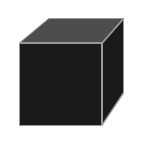• sphere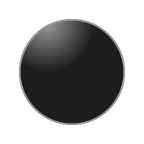• cylinder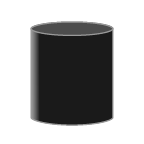• cone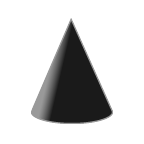• torus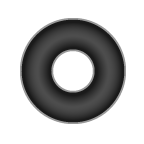All data points of one shapeCloud plot have the same shape. You can combine multiple shape plots with different shapes. If legendShowShape is set to TRUE, the plot will be listed in a separate legend, with its name and shape.

Additionally, each data point can have an information string assigned to it that is displayed when the corresponding shape in the plot is clicked. These strings can include line breaks ("\n").

The shapeCloud() plotting function takes the following arguments:

Argument Type Default Description
coords matrix required 3 column matrix with x, y and z coordinates.
colorBy character required Controls how the colorVar values are interpreted. Either "categories" for discrete values, "values" for continuous values, or "direct" when colorVar is a vector of colors in hex format (e.g. "#e95420").
colorVar character or numerical vector required Color variable for the data points, containing category names, numerical values, or hex color strings, depending on the colorBy parameter.
name character "shape cloud" Name of the plot for displaying it in a plot shape legend.
shape character "box" Shape of the data points in the plot. One of c("box", "sphere", "torus", "cone", "cylinder").
size double 1 Point size
width integer NULL Width of the widget, fits to the viewer pane when left empty.
height integer NULL Height of the widget, fits to the viewer pane when left empty.
xScale double 1 Scale x axis by a factor.
yScale double 1 Scale y axis by a factor
zScale double 1 Scale z axis by a factor
colorScale character NULL Name of a colorbrewer scale (e.g. "OrRd"), "Viridis", or "custom". If set to "custom", the customColorScale parameter is also needed.
customColorScale character vector NULL Character vector of at least 2 colors. Only needed if colorScale is set to "custom".
sortedCategories character vector NULL Optional character vector with all category names if colorBy is "categories", to sort the categories in the legend and to the colorscale.
backgroundColor character "#ffffffff" Color of background as hex color string.
showLegend logical TRUE Display legend for categories of values.
showShape logical TRUE Add this plot to the legend of plot shapes.
fontSize double 11 Size of legend text.
fontColor character "black" Color of legend text. As a valid html color (e.g. "black" or "#000000").
legendTitle character "" Title for the legend
legendTitleFontSize double 16 Size of the legend title font.
legendTitleFontColor character "black" Color of the legend title font.
showAxes logical or list FALSE Display plot axes. Either one logical, or a list of three logicals, one for each axis (x, y, z).
axisLabels list list("X", "Y", "Z") List of labels for the axes.
axisColors character or list "#666666" Colors for the axes and their labels. Either one hex string (e.g. #000000), or a list of three hex strings, one for each axis (x, y, z).
tickBreaks double or list 2 Interval of ticks on axes. Either one numerical value, or a list of three numbers, one for each axis (x, y, z).
showTickLines logical, list or list of lists FALSE Display lines at tick intervals along axes. Either one logical, a list of three logicals, one for each axis (x, y, z), or a list of three lists with two logicals each, for each axis plane ([xy, xz], [yx, yz], [zx, zy]).
tickLineColors character, list or list of lists "#aaaaaa" Colors for the tick lines. Either one hex string (e.g. #000000), a list of three strings, one for each axis (x, y, z), or a list of three lists with two strings, for each axis plane ([xy, xz], [yx, yz], [zx, zy]).
folded logical FALSE Show animation of 2D to 3D point cloud.
foldedEmbedding matrix NULL A 2D embedding of the 3D data to be used for the unfolding animation. 2 column matrix.
foldAnimDelay integer 100 Number of frames before animation from 2D to 3D is started.
foldAnimDuration integer 200 Number of frames for transitioning between 2D and 3D.
dpInfo character vector NULL Vector with information to be displayed for each data point on click.
turntable logical FALSE Rotate the camera around the plot.
rotationRate double 0.01 Speed of turntable rotation. A positive value rotates the camera counter clockwise, a negative value clockwise.
shapeLegendTitle character "" Title for the plot shape legend.
upAxis character "+y" Sets the camera up vector; Either "+x", "-x", "+y", "-y", "+z", or "-z".
showUI logical FALSE Display buttons on the visualization. These buttons make it possible to add labels to the plot, or to export the plot as a JSON file.

#### Surface

The surface plot creates a 3 dimensional surface that is defined by a height variable for each point on a x-y plane given by the rows and columns of a 2d matrix. An additional variable can be visualized by the color of the surface points. The color is interpolated between the defined points of the surface.

The surface() plotting function takes the following arguments:

Argument Type Default Description
coords matrix required 2-dimensional matrix with height data.
colorBy character required Controls how the colorVar values are interpreted. Either "categories" for discrete values, "values" for continuous values, or "direct" when colorVar is a vector of colors in hex format (e.g. "#e95420").
colorVar character or numerical vector required Color variable for the data points, containing category names, numerical values, or hex color strings, depending on the colorBy parameter.
name character "shape cloud" Name of the plot for displaying it in a plot shape legend.
width integer NULL Width of the widget, fits to the viewer pane when left empty.
height integer NULL Height of the widget, fits to the viewer pane when left empty.
xScale double 1 Scale x axis by a factor.
yScale double 1 Scale y axis by a factor
zScale double 1 Scale z axis by a factor
colorScale character NULL Name of a colorbrewer scale (e.g. "OrRd"), "Viridis", or "custom". If set to "custom", the customColorScale parameter is also needed.
customColorScale character vector NULL Character vector of at least 2 colors. Only needed if colorScale is set to "custom".
backgroundColor character "#ffffffff" Color of background as hex color string.
showLegend logical TRUE Display legend for categories of values.
showShape logical FALSE Add this plot to the legend of plot shapes.
fontSize double 11 Size of legend text.
fontColor character "black" Color of legend text. As a valid html color (e.g. "black" or "#000000").
legendTitle character "" Title for the legend
legendTitleFontSize double 16 Size of the legend title font.
legendTitleFontColor character "black" Color of the legend title font.
showAxes logical or list FALSE Display plot axes. Either one logical, or a list of three logicals, one for each axis (x, y, z).
axisLabels list list("X", "Y", "Z") List of labels for the axes.
axisColors character or list "#666666" Colors for the axes and their labels. Either one hex string (e.g. #000000), or a list of three hex strings, one for each axis (x, y, z).
tickBreaks double or list 2 Interval of ticks on axes. Either one numerical value, or a list of three numbers, one for each axis (x, y, z).
showTickLines logical, list or list of lists FALSE Display lines at tick intervals along axes. Either one logical, a list of three logicals, one for each axis (x, y, z), or a list of three lists with two logicals each, for each axis plane ([xy, xz], [yx, yz], [zx, zy]).
tickLineColors character, list or list of lists "#aaaaaa" Colors for the tick lines. Either one hex string (e.g. #000000), a list of three strings, one for each axis (x, y, z), or a list of three lists with two strings, for each axis plane ([xy, xz], [yx, yz], [zx, zy]).
turntable logical FALSE Rotate the camera around the plot.
rotationRate double 0.01 Speed of turntable rotation. A positive value rotates the camera counter clockwise, a negative value clockwise.
shapeLegendTitle character "" Title for the plot shape legend.
upAxis character "+y" Sets the camera up vector; Either "+x", "-x", "+y", "-y", "+z", or "-z".
showUI logical FALSE Display buttons on the visualization. These buttons make it possible to add labels to the plot, or to export the plot as a JSON file.

#### Heat map

The heat map is a 3 dimensional bar chart defined similarly to the surface plot, where the height of each bar on a x-y plane is given by the value in a row-column pair of a 2d matrix. An additional variable can be visualized by the color of the bar.

The heatMap() plotting function takes the following arguments:

Argument Type Default Description
coords matrix required 2-dimensional matrix with height data.
colorBy character required Controls how the colorVar values are interpreted. Either "categories" for discrete values, "values" for continuous values, or "direct" when colorVar is a vector of colors in hex format (e.g. "#e95420").
colorVar character or numerical vector required Color variable for the data points, containing category names, numerical values, or hex color strings, depending on the colorBy parameter.
name character "shape cloud" Name of the plot for displaying it in a plot shape legend.
size double 1 Controls the width in the x and z dimensions of the pillars. Values should be between 0 and 1, values over 1 cause the pillars to intersect each other.
width integer NULL Width of the widget, fits to the viewer pane when left empty.
height integer NULL Height of the widget, fits to the viewer pane when left empty.
xScale double 1 Scale x axis by a factor.
yScale double 1 Scale y axis by a factor
zScale double 1 Scale z axis by a factor
colorScale character NULL Name of a colorbrewer scale (e.g. "OrRd"), "Viridis", or "custom". If set to "custom", the customColorScale parameter is also needed.
customColorScale character vector NULL Character vector of at least 2 colors. Only needed if colorScale is set to "custom".
backgroundColor character "#ffffffff" Color of background as hex color string.
showLegend logical TRUE Display legend for categories of values.
showShape logical FALSE Add this plot to the legend of plot shapes.
fontSize double 11 Size of legend text.
fontColor character "black" Color of legend text. As a valid html color (e.g. "black" or "#000000").
legendTitle character "" Title for the legend
legendTitleFontSize double 16 Size of the legend title font.
legendTitleFontColor character "black" Color of the legend title font.
showAxes logical or list FALSE Display plot axes. Either one logical, or a list of three logicals, one for each axis (x, y, z).
axisLabels list list("X", "Y", "Z") List of labels for the axes.
axisColors character or list "#666666" Colors for the axes and their labels. Either one hex string (e.g. #000000), or a list of three hex strings, one for each axis (x, y, z).
tickBreaks double or list 2 Interval of ticks on axes. Either one numerical value, or a list of three numbers, one for each axis (x, y, z).
showTickLines logical, list or list of lists FALSE Display lines at tick intervals along axes. Either one logical, a list of three logicals, one for each axis (x, y, z), or a list of three lists with two logicals each, for each axis plane ([xy, xz], [yx, yz], [zx, zy]).
tickLineColors character, list or list of lists "#aaaaaa" Colors for the tick lines. Either one hex string (e.g. #000000), a list of three strings, one for each axis (x, y, z), or a list of three lists with two strings, for each axis plane ([xy, xz], [yx, yz], [zx, zy]).
folded logical FALSE Show animation of 2D to 3D point cloud.
foldedEmbedding matrix NULL A 2D embedding of the 3D data to be used for the unfolding animation. 2 column matrix.
foldAnimDelay integer 100 Number of frames before animation from 2D to 3D is started.
foldAnimDuration integer 200 Number of frames for transitioning between 2D and 3D.
turntable logical FALSE Rotate the camera around the plot.
rotationRate double 0.01 Speed of turntable rotation. A positive value rotates the camera counter clockwise, a negative value clockwise.
shapeLegendTitle character "" Title for the plot shape legend.
upAxis character "+y" Sets the camera up vector; Either "+x", "-x", "+y", "-y", "+z", or "-z".
showUI logical FALSE Display buttons on the visualization. These buttons make it possible to add labels to the plot, or to export the plot as a JSON file.

#### Line plot

Lines are 3 dimensional continuous lines that are described by the x, y and z coordinates of their vertices. Each point can also have a color variable which will be interpolated on the line connecting the points.

The linePlot() plotting function takes the following arguments:

Argument Type Default Description
coords matrix required 3 column matrix with x, y and z coordinates.
colorBy character required Controls how the colorVar values are interpreted. Either "categories" for discrete values, "values" for continuous values, or "direct" when colorVar is a vector of colors in hex format (e.g. "#e95420").
colorVar character or numerical vector required Color variable for the data points, containing category names, numerical values, or hex color strings, depending on the colorBy parameter.
name character "point cloud" Name of the plot for displaying it in a plot shape legend.
width integer NULL Width of the widget, fits to the viewer pane when left empty.
height integer NULL Height of the widget, fits to the viewer pane when left empty.
xScale double 1 Scale x axis by a factor.
yScale double 1 Scale y axis by a factor
zScale double 1 Scale z axis by a factor
colorScale character NULL Name of a colorbrewer scale (e.g. "OrRd"), "Viridis", or "custom". If set to "custom", the customColorScale parameter is also needed.
customColorScale character vector NULL Character vector of at least 2 colors. Only needed if colorScale is set to "custom".
sortedCategories character vector NULL Optional character vector with all category names if colorBy is "categories", to sort the categories in the legend and to the colorscale.
labels character vector NULL A list or vector of labels to be displayed alongside the line points.
labelSize double 100 Size of the point labels.
labelColor character "match" Color of the labels. Can also be "match", in which case the label takes the color of the corresponding point.
backgroundColor character "#ffffffff" Color of background as hex color string.
showLegend logical TRUE Display legend for categories of values.
showShape logical FALSE Add this plot to the legend of plot shapes.
fontSize double 11 Size of legend text.
fontColor character "black" Color of legend text. As a valid html color (e.g. "black" or "#000000").
legendTitle character "" Title for the legend
legendTitleFontSize double 16 Size of the legend title font.
legendTitleFontColor character "black" Color of the legend title font.
showAxes logical or list FALSE Display plot axes. Either one logical, or a list of three logicals, one for each axis (x, y, z).
axisLabels list list("X", "Y", "Z") List of labels for the axes.
axisColors character or list "#666666" Colors for the axes and their labels. Either one hex string (e.g. #000000), or a list of three hex strings, one for each axis (x, y, z).
tickBreaks double or list 2 Interval of ticks on axes. Either one numerical value, or a list of three numbers, one for each axis (x, y, z).
showTickLines logical, list or list of lists FALSE Display lines at tick intervals along axes. Either one logical, a list of three logicals, one for each axis (x, y, z), or a list of three lists with two logicals each, for each axis plane ([xy, xz], [yx, yz], [zx, zy]).
tickLineColors character, list or list of lists "#aaaaaa" Colors for the tick lines. Either one hex string (e.g. #000000), a list of three strings, one for each axis (x, y, z), or a list of three lists with two strings, for each axis plane ([xy, xz], [yx, yz], [zx, zy]).
turntable logical FALSE Rotate the camera around the plot.
rotationRate double 0.01 Speed of turntable rotation. A positive value rotates the camera counter clockwise, a negative value clockwise.
shapeLegendTitle character "" Title for the plot shape legend.
upAxis character "+y" Sets the camera up vector; Either "+x", "-x", "+y", "-y", "+z", or "-z".
showUI logical FALSE Display buttons on the visualization. These buttons make it possible to add labels to the plot, or to export the plot as a JSON file.

#### Image stack

The image stack visualizes a set of 2 dimensional slices with pixel values. The input is a tiff stack with rgb channels. It was created and optimized for fluorescent microscopy z-stack data and therefore works best with black background and bright signal.

The imgStack() plotting function takes the following arguments:

Argument Type Default Description
img character required Path to RGB tiff image stack.
size double 1 Point size
threshold double or list 0.1 Intensity threshold for background removal. Either one number (between 0 and 1) or list of numbers with threshold for each channel. (default 0.1).
width integer NULL Width of the widget, fits to the viewer pane when left empty.
height integer NULL Height of the widget, fits to the viewer pane when left empty.
xScale double 1 Scale x axis by a factor.
yScale double 1 Scale y axis by a factor
zScale double 1 Scale z axis by a factor
backgroundColor character "#ffffffff" Color of background as hex color string.
intensityMode character "alpha" Representation of pixel intensity, either "none", "alpha" or "mix". The default "alpha" generally looks best, but might be slightly slower than the other two options.
channelColors list list("#ff0000", "#00ff00", "#0000ff") Set the colors for each of the channels, the default is for RGB images.
channelOpacities list list(1, 1, 1) Set an overall opacity for each channel (default: list(1, 1, 1)). Only works together with intensityMode "alpha".
invertIntensities logical FALSE Invert pixel intensity values. Set TRUE if image has dark foreground and light background. Inversion happens before thresholding.
showAxes logical or list FALSE Display plot axes. Either one logical, or a list of three logicals, one for each axis (x, y, z).
axisLabels list list("X", "Y", "Z") List of labels for the axes.
axisColors character or list "#666666" Colors for the axes and their labels. Either one hex string (e.g. #000000), or a list of three hex strings, one for each axis (x, y, z).
tickBreaks double or list 2 Interval of ticks on axes. Either one numerical value, or a list of three numbers, one for each axis (x, y, z).
showTickLines logical, list or list of lists FALSE Display lines at tick intervals along axes. Either one logical, a list of three logicals, one for each axis (x, y, z), or a list of three lists with two logicals each, for each axis plane ([xy, xz], [yx, yz], [zx, zy]).
tickLineColors character, list or list of lists "#aaaaaa" Colors for the tick lines. Either one hex string (e.g. #000000), a list of three strings, one for each axis (x, y, z), or a list of three lists with two strings, for each axis plane ([xy, xz], [yx, yz], [zx, zy]).
turntable logical FALSE Rotate the camera around the plot.
rotationRate double 0.01 Speed of turntable rotation. A positive value rotates the camera counter clockwise, a negative value clockwise.
upAxis character "+y" Sets the camera up vector; Either "+x", "-x", "+y", "-y", "+z", or "-z".
showUI logical FALSE Display buttons on the visualization. These buttons make it possible to add labels to the plot, or to export the plot as a JSON file.

#### Mesh object

The meshObject visualizes a 3D model, or a scene of multiple 3D models from a glTF file.

The meshObject() plotting function takes the following arguments:

Argument Type Default Description
filepath character required Path to RGB glTF file.
meshScaling list list(1, 1, 1) List of three numbers to scale the object(s) along the axes.
meshRotation list list(0, 0, 0) List of three numbers to rotate the object(s) along the axes.
meshOffset list list(0, 0, 0) List of three numbers to offset the position of the object(s) along the axes.
width integer NULL Width of the widget, fits to the viewer pane when left empty.
height integer NULL Height of the widget, fits to the viewer pane when left empty.
xScale double 1 Scale x axis by a factor.
yScale double 1 Scale y axis by a factor
zScale double 1 Scale z axis by a factor
backgroundColor character "#ffffffff" Color of background as hex color string.
showAxes logical or list FALSE Display plot axes. Either one logical, or a list of three logicals, one for each axis (x, y, z).
axisLabels list list("X", "Y", "Z") List of labels for the axes.
axisColors character or list "#666666" Colors for the axes and their labels. Either one hex string (e.g. #000000), or a list of three hex strings, one for each axis (x, y, z).
tickBreaks double or list 2 Interval of ticks on axes. Either one numerical value, or a list of three numbers, one for each axis (x, y, z).
showTickLines logical, list or list of lists FALSE Display lines at tick intervals along axes. Either one logical, a list of three logicals, one for each axis (x, y, z), or a list of three lists with two logicals each, for each axis plane ([xy, xz], [yx, yz], [zx, zy]).
tickLineColors character, list or list of lists "#aaaaaa" Colors for the tick lines. Either one hex string (e.g. #000000), a list of three strings, one for each axis (x, y, z), or a list of three lists with two strings, for each axis plane ([xy, xz], [yx, yz], [zx, zy]).
turntable logical FALSE Rotate the camera around the plot.
rotationRate double 0.01 Speed of turntable rotation. A positive value rotates the camera counter clockwise, a negative value clockwise.
upAxis character "+y" Sets the camera up vector; Either "+x", "-x", "+y", "-y", "+z", or "-z".
showUI logical FALSE Display buttons on the visualization. These buttons make it possible to add labels to the plot, or to export the plot as a JSON file.

### The babyplots object

All plotting functions create a babyplots object. When printed to the console, this object shows the visualization. Assigning it to a variable allows you to change parameters of the visualization after its creation using the $ selector. The babyplots object has the following slots:  Slot Type Description plots list A list of all plots that have been added to this babyplots object. The plots themselves are named lists, so their parameters that were set at the creation can also be accessed and changed through this slot. turntable logical Controls the camera rotation around the plot rotationRate double The speed at which the camera rotates around the plot, if turntable is set to TRUE shapeLegendTitle character "" Title for the plot shape legend. showUI logical Display buttons on the visualization. backgroundColor character Color of background as hex color string. xScale double Scale x axis by a factor. yScale double Scale y axis by a factor zScale double Scale z axis by a factor Adding a two babyplots objects together creates a new babyplots object that contains both plots in the $plot slot. The general options (e.g. turntable, showUI, ...) are carried over from the first babyplots object.